SEARCH HOMEMath Central Quandaries & QueriesQuestion from Rogerson, a student: Find the area, S, enclosed by the curve y = -x^2 + 6x - 5 and the x-axis in the interval 0≤x≤4. thanks.Rogerson,

I graphed the function y = -x2 - 6x - 5 for -2 ≤ x ≤ 4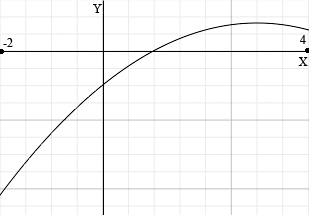I then erased everything but the curve and the x-axis for 0 ≤ x ≤ 4.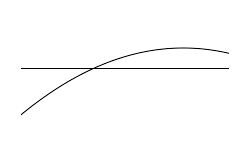As you can see there is no finite region bounded by these two curves. Are you sure you have the question worded correctly?

Harley

Rogerson wrote back

Question from Rogerson, a student:

About my last question, yes I'm sure I worded it correctly. I even checked the problem and it is correct. in fact here is a similar question. If you can answer, thanks a lot.

Find the area, S, enclosed by the curve y = x^2 + 4x and the x-axis in the interval -1 ≤ x ≤ 4.

I think the question should say

Find the area, S, between the curve y = -x2 + 6x - 5 and the x-axis in the interval 0≤x≤4.

I solved y = -x2 + 6x - 5 and y = 0 to find that the curve intersects the x-axis at 1 nd 5. T then redrew my first diagram and shaded the region between the curve and the x-axis for 0 ≤ x ≤ 4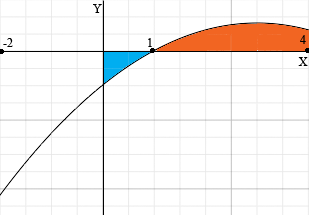Part of the region is above the x-axis and part is below the x-axis. For the part above the x-axis, the part with 1 ≤ x ≤ 4, the area is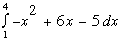A similar integral from 0 to 1 will give a negative value since in that range the curve is below the x-axis. Hence you need to change the sign of the integral to obtain the area above the curve and below the x-axis. Thus the area, S, between the curve y = -x2 + 6x - 5 and the x-axis in the interval 0≤x≤4 is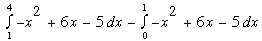Evaluate this and try the second problem you sent. If you need further help write back.

HarleyMath Central is supported by the University of Regina and The Pacific Institute for the Mathematical Sciences.